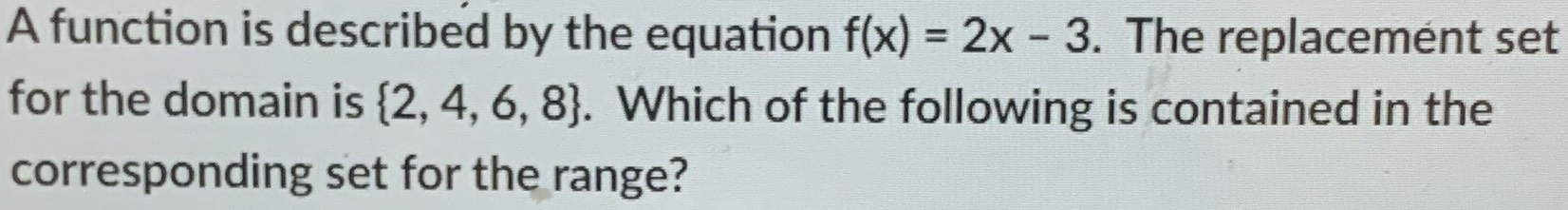### ¿Todavía tienes preguntas de matemáticas?

Pregunte a nuestros tutores expertos
Algebra
PreguntaA function is described by the equation $$f ( x ) = 2 x - 3$$ . The replacement set for the domain is $$\{ 2,4,6,8 \}$$ . Which of the following is contained in the corresponding set for the range?